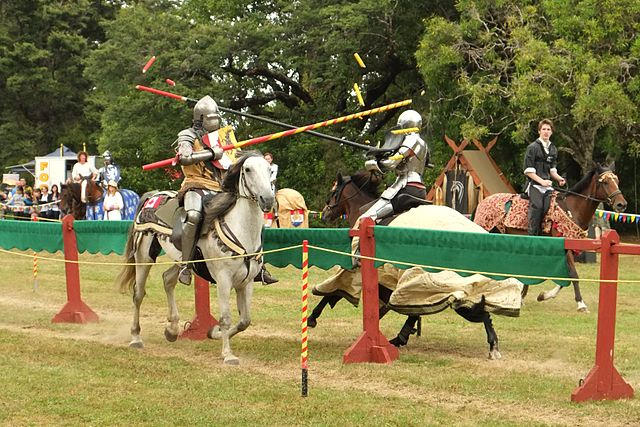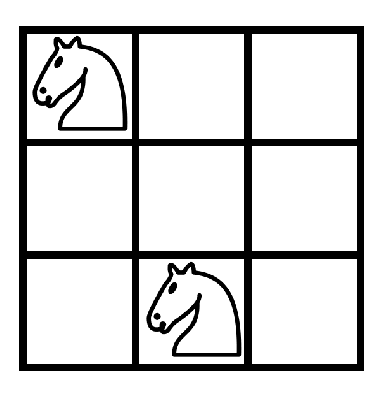# The Knights Dilemma

Probability Level 3What is the maximum number of knights you can place on a $8 \times 8$ chessboard such that no two of them attack each other?

Details and assumptions

• The knights are placed on distinct cells.

• Two knights attack each other if they are one cell vertically and two cells horizontally apart or two cells vertically and one cell horizontally apart.

• A $8 \times 8$ chessboard has $8^2= 64$ cells.

• Here's an example of $2$ knights being placed on a $3 \times 3$ chessboard which attack each other.×